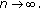• Research Article
• Open Access

# A Limit Theorem for the Moment of Self-Normalized Sums

Journal of Inequalities and Applications20092009:957056

https://doi.org/10.1155/2009/957056

• Received: 25 December 2008
• Accepted: 18 June 2009
• Published:

## Abstract

Letbe a sequence of independent and identically distributed (i.i.d.) random variables andis in the domain of attraction of the normal law and. For, we prove the precise asymptotics in Davis law of large numbers for## Keywords

• Limit Theorem
• Triangle Inequality
• Significant Development
• Asymptotic Normality
• Strong Approximation

## 1. Introduction and Main Result

Throughout this paper, we letbe a sequence ofrandom variables andis in the domain of attraction of the normal law and. Put
Also letThen by the well-known Davis laws of large numbers ,

if and only ifand.

Gut and Spătaru  proved its precise asymptotics as follows.

Theorem 1 A.

Suppose thatandThen for,

wherestands for theabsolute moment of the standard normal distribution.

It is well known that, forrandom variables, Chow  discussed the complete moment convergence, and got the following result.

Theorem 1 B.

Letbe a sequence ofrandom variables with. Assume,, andThen for any,

On the other hand, the past decade has witnessed a significant development on the limit theorems for the so-called self-normalized sum. Bentkus and Götze  obtained Berry-Esseen inequalities for self-normalized sums. Wang and Jing  derived exponential nonuniform Berry-Esseen bound. Giné et al. , established asymptotic normality of self-normalized sums.

Theorem 1 C.

Letbe a sequence ofrandom variables with. Then for any,

holds, if and only ifis in the domain of attraction of the normal law, whereis the distribution function of the standard normal random variable.

Shao  showed a self-normalization large deviation result forwithout any moment conditions.

Theorem 1 D.

Letbe a sequence of positive numbers withandas. Ifandis slowly varying asthen

Since then, many subsequent developments of self-normalized sums have been obtained. For example, Csörgő et al.  have established Darling-Erdös theorem for self-normalized sums, and they  have also obtained Donsker's theorem for self-normalized partial sums processes.

Inspired by the above results, in this note we study the precise asymptotics in Davis law of large numbers for the moment of self-normalized sums. Our main result is as follows.

Theorem 1.1.

Supposeis in the domain of attraction of the normal law and. Then, forand, one has

here and in the sequel,is the standard normal random variable.

Remark 1.2.

Ifand, by the strong law of large numbers, we haveThen, we can easily obtain the following result:

Remark 1.3.

As is well known, the strong approximation method is taken in order to obtain such an analogous result, however, this method is not applicable here.

## 2. Proof of Theorem 1.1

In this section, we setforand. Here and in the sequel,will denote positive constants, possibly varying from place to place, andmeans the largest integer. The proof of Theorem 1.1 is based on the following propositions.

Proposition 2.1.

For, one has

Proof.

Via the change of variable, we have

Proposition 2.2.

For, one has

Proof.

SetThen, by (1.5), it is easy to seeasObserve that
Thus for, it is easy to see
Now we are in a position to estimate. From (1.6), and by applyingto it, we can obtain that for large enoughand any, there exist C and b such thatfor. In particular, for, there existssuch that
For, by Markov's inequality and (2.7), we have
Denote, then, since the weighted average of a sequence that converges to 0 also converges to 0, it follows that, for any,

The proof is completed.

Proposition 2.3.

For, one has

Proof.

So this proposition is proved now.

Proposition 2.4.

For, one has

Proof.

For, by (2.7), we have
For, using (2.7) again, we have

Combining (2.18), (2.19), and (2.20), the proposition is proved.

Our main result follows from the propositions using the triangle inequality.

## Declarations

### Acknowledgments

The author thanks the referees for pointing out some errors in a previous version, as well as for several comments that have led to improvements in this work. Thanks are also due to Doctor Ke-ang Fu of Zhejiang University in china for his valuable suggestion in the preparation of this paper.

## Authors’ Affiliations

(1)
Department of Mathematics, Huaiyin Teachers College, Huaian, 223300, China

## References